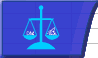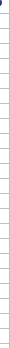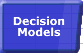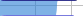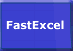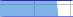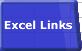# Excel Calculation ProcessSmart Recalculation Evaluation Circumstances Calculation Process Dependency Trees Controlling Calculation "CALCULATE" in Status Bar Calculation Methods Calculating from VBA Volatile Functions & Actions User-Defined Functions Repetitive Calculation Version Timing ComparisonCalculation Process Dependencies Forward References Cell Calculation Sequence Worksheet Sequence Multi-threaded Calculation

## Excel's Calculation Process

Excel does not calculate cells in a fixed order, or by Row or Column. Instead, Excel dynamically determines and remembers its own internal calculation sequence, which is based on dependencies and dependency trees and chains.

Overall Excel has a two step calculation process:

• Step 1: Build Dependency Trees and flag cells as uncalculated.
• Step 2: Determine the dependency tree calculation sequence, calculate the uncalculated formulae according to the calculation sequence, and remember the calculation sequence for the next recalculation.

The first step is executed at each cell entry or change even in Manual calculation mode. Normally this executes so fast that you do not notice it happening, but if there is a noticeable delay in Manual mode then in Excel 2007 setting Workbook.ForceFullCalculation to true will eliminate the delay at the expense of making each calculation a Full calculation.

The second step is executed at each calculation or recalculation.
In Excel 2007 individual branches of the calculation tree can be assigned to multiple threads which, if you have multiple processors, can be calculated in parallel. This can significantly speed up calculation particularly on large models.
Note that the dependency tree calculation process continues even when the value of a cell that has been calculated does not change.

The second time you calculate a workbook is often significantly faster than the first. This is due to several factors:

• Excel usually only recalculates cells which have changed, and their dependents.
• Excel stores and reuses the most recent calculation sequence so can save most of the time taken to determine the calculation sequence.
• Within an Excel session both Windows and Excel will cache recently used data and programs for faster access.

#### Dependencies

 Dependencies control the way cells are flagged as uncalculated, and the final sequence they must be calculated in. Unreferenced dependencies in functions can give you unexpected (wrong) answers. When a cell in a spreadsheet refers to another cell it must be finally calculated after the cell it refers to. This is called a Dependency. Excel recognizes dependencies by looking at each formula and seeing what cells are referred to. See Dependency Trees for more details of how Excel determines dependencies. Understanding this is important for User Defined Functions because you need to make sure that all the cells the function uses are referred to in the function arguments. Otherwise Excel may not be able to correctly determine when the function needs to calculated, and what its dependencies are, and you may get an unexpected answer. Specifying Application.Volatile or using Ctrl/Alt/F9 will often enable Excel to bypass this problem, but you still need to write your function to handle multiple executions per calculation cycle and uncalculated data. See the separate page on User-Defined Functions for further explanation.

#### Forward References

 A Forward Reference refers to a cell that has not yet been calculated. A dependency can be either a Backward Dependency/Backward Reference (refers to a cell that has already been finally calculated) or a Forward Dependency/Forward Reference (refers to a cell that has not yet been finally calculated) These dependencies are called: Within Sheet Dependencies/References when a cell refers to a cell on the same Worksheet. Inter Sheet Dependencies/References when a cell refers to a cell on another Worksheet in the same Workbook. Inter Workbook Dependencies/References when a cell refers to a cell in another Workbook. For example: A1=A2+5 A2=A3+1 A3=2 These cells have to be finally calculated in the order A3 A2 A1 Cell A1 Forward References cell A2 and cell A2 Forward References cell A3.

#### Cell Calculation Sequence.

 Excels calculation sequence is dynamic, and may change from calculation to calculation. Sometimes Excel will decide that the fastest way to calculate the workbook involves calculating a particular cell more than once. When Excel calculates a Worksheet it uses an iterative process of calculating cells to discover the correct calculation sequence, which it will then use in subsequent recalculations. Some actions such as Range Calculate can reset the internal calculation sequence: Selecting a single rectangular range and doing a Range Calculate will reset the calculation sequence of all the formulae within the rectangular range to left-to-right, top-to-bottom in Excel 97 and 2000. If there are depencies between cells within the range then Excel2002 and 2003 will reset the calculation sequence of all the formulae in the rectangular range to dependency calculation sequence for the range. If more than one area is selected, each area is calculated left-to-right and top-to-bottom, in the sequence that the areas were selected. The time taken by Excel to work out the calculation sequence can be increased by heavy use of functions such as INDIRECT and OFFSET which increase the complexity and volatility of the dependency tree. Comparing the time taken for a Range Calculate for the used range on a worksheet with the time taken for a Sheet Calculate immediately after the Range Calculate will give you a measurement of the time taken to determine the optimum calculation sequence for a worksheet. If you do the Range Calculate followed by two Sheet Calculates a comparison of the second Sheet Calculate with the first will give you a measurement of the volatility of the formulae and the dependency tree.

The default calculation method for Excel is to only calculate cells that are flagged as either uncalculated or dependent on an uncalculated cell.
Excel will still use its internal dynamic calculation sequence, but will only calculate the flagged cells in the sequence.

 Excelss initial calculation sequence is to evaluate the most recently entered uncalculated cells first. The second calculation starts by using the most recent calculation sequence. The default initial calculation sequence for Excel is to evaluate cells in the reverse sequence in which formulae were entered/changed on a sheet (most recently entered first, or LIFO), and then to modify this sequence as required by the dependency trees. The final calculation sequence is determined so that all cells in dependency chains are calculated in the correct order. For most Excel formulae and functions this process of sorting the cells into dependency chain sequence is relatively fast, and the dependency chain sequence is stored after each calculation so that subsequent recalculations are even faster. If the time taken by a Range.Calculate for all the formulae on a sheet is significantly less than the time taken by a Sheet.Calculate, then the organisation and volatility of the formulae on the sheet may be causing a bottleneck in determining the dependency chain sequence. For User Defined Functions Excel has to execute the function in order to determine if it contains hidden dependencies in references to cells which are not in the argument list. Note that if you put a false dependency in the argument list (a reference which is not actually used inside the function) Excel will execute the function, but not neccessarily in the sequence you expect. Also Excel will not recognise a dependency that is bypassed by an IF statement. These factors can cause problems in your UDF unless you take the appropriate precautions.

#### Circular Reference Cell Calculation Sequence

When your workbook contains circular references and Iteration is switched on (Application.Iteration is True) Excel has to use a slightly different calculation algorithm:

The first step is the same as a recalculation (or full calculation, depending how the calculation was invoked) that does not involve circular references. This enables all the cells that come before the circular reference to be properly calculated and the list of cells in the circular reference to be identified together with their dependents. During this first step Application.Iteration is False.

The second step occurs once on each iteration:

• The list of cells in the circular reference is calculated sheet by sheet, in alphabetic sheet name sequence (even in Excel 2002 & 2003). Within each sheet the list of cells and array formulae in the circular reference, and all cells that are dependent on them, is calculated from left to right and top to bottom. (all the columns in the first row from left to right, then the next row ...). Each array formula in the list is calculated as a single block of cells from left to right and top to bottom, so if you want to use calculation by columns rather than by rows you have to use a multi-cell column array formula.
• This calculation ignores the dependency tree and relies on the iterative calculation process to resolve dependencies.
• Volatile cells that are not dependent on the cells in the circular list are NOT recalculated on each iteration.

This step is repeated until the iteration limits (maximum number of iterations and maximum cell change per iteration) are reached. During this second step Application.Iteration is True.

#### Data Validation and Automatic Calculation

When you are using Data Validation rules on cells with Automatic Calculation the result of the data validation may be different if Recalculation is done before data validation, or data validation is done before Recalculation.

Experiments show that this sequence is dependent on the way you exit the cell after changing the data.
Clicking another cell causes the data validation to be done before the recalculation
.
Using Tab or the Arrow keys or Enter to exit the cell causes the data validation to be done after the calculation .

#### Microsoft Calculation Documentation

Some more details of Excel's calculation algorithm and the changes made in Excel 2002 have recently been published by Microsoft.

#### Workbook and Worksheet calculation sequence.

 Excel97 & 2000 calculate Worksheets in alphabetic name sequence. A separate calculation sequence list is maintained for each sheet. Excel97 and Excel2000 calculate Workbooks, and Worksheets within each Workbook, in alphabetic name sequence: Book1 calculates before Book2. Within a Workbook Balance Sheet calculates before Cash Flow, which calculates before Profit Loss, regardless of the visible order of the Worksheet Tabs in the Workbook. A separate dependency chain calculation sequence is developed for each sheet, together with a calculation flag for each sheet and each book. Significant calculation speed gains can often be made by determining the optimum worksheet naming sequence. See the FastExcel V4 CrossRefs command. Excel2002/Excel2003 also calculates workbooks and worksheets in alphabetic sequence but develops a single global dependency chain sequence for all open workbooks. For complex workbooks containing many forward worksheet references and/or circular worksheet references using Excel 2002 can significantly speed up recalculation times. However because the global dependency chain can be a lot larger than the individual dependency chains for each worksheet the initial calculation time to determine the correct dependency tree calculation sequence can sometimes be significantly greater than it was with Excel 97/2000. (see http://www.whooper.co.uk/excelstuff.htm for an example problem that demonstrates this effect). If this effect becomes a problem you may be able to bypass it in Manual mode by resetting the initial calculation sequence of each sheet using UsedRange.calculate. Excel95 and Excel5 calculate Worksheets in the sequence in which they are defined. (Thanks to Rob Bovey for this tip).

Excel 2007 can split calculation across multiple processors or cores. When Excel 2007 loads a workbook, it determines from the operating system how many processors are available and then creates a separate calculation thread for each processor. These threads can then run in parallel. The beauty of this system is that it scales extremely well with the number of processors.

Most workbooks show a significant improvement in calculation speed on a system with multiple cores. The degree of improvement depends on how many independent calculation trees the workbook contains. If you make a workbook that contains one continuous chain of formulas, it will not show any multithreaded calculation (MTC) performance gain, whereas a workbook that contains several independent chains of formulas will show gains close to the number of processors available.

A test on a range of workbooks with between 840K and 23K formulas using Excel 2007 on a Dual-Core system showed improvement factors from using MTC ranging from 1.9 to no improvement, with the larger workbooks tending to show the most improvement.

In collaboration with Intel Corporation, Microsoft conducted testing on a suite of user-created spreadsheets. Comparisons were done between Excel 2007 and Excel 2003. (A prerelease version of Excel 2007 was used, but little to no difference is expected with the final release version.)

Results showed calculation times ranging from no improvement to greater than theoretical (2x/4x) improvement on both the Dual-Core and Quad-Processor systems. Typical (median) improvement for a system with an Intel Dual-Core Pentium 4 at 3.0 GHz with 1 GB of RAM compared to the same file calculating in Excel 2003 were 48 percent, or a 1.92x speedup. Typical (median) speedup for a system with an Intel Quad-Core Xeon at 3.0 GHz with 4 GB of RAM were 76 percent, or a 4.17x speedup. Similar speed improvements were observed on other processors and platforms. Improvements beyond theoretical speedup (due to multithreading) are attributed to other performance enhancements in Excel 2007, such as enhancements to the speed of function execution.

Some Excel features do not use multithreaded calculation, for example:

• Data table calculation (but structured references to tables do use MTC).
• User-defined functions (but XLL functions can be multithread-enabled).
• XLM functions.
• INDIRECT, CELL functions that use either the format2 or address options.
• GETPIVOTDATA and other functions referring to PivotTables or cubes.
• Range.Calculate and Range.CalculateRowMajorOrder.
• Cells in circular reference loops.

The first time that Excel calculates a workbook on a computer that has multiple processors, you incur some overhead while Excel examines dependencies. Therefore, you can see the maximum performance increase on the second and subsequent calculations (although there is still usually improvement on the first calculation versus running the same task on the same speed of computer with a single processor).

You also incur this overhead the first time you calculate a workbook on a multiple-processor computer that has a larger number of processors than the computer on which you last saved the workbook. If you turn off MTC, or run Excel 2007 on a system that has a single processor, there is no performance gain or loss from the MTC feature. You can use MTC in Excel 2007 even in compatibility mode, and the information that is stored by the calculation can be reused even after the workbook is calculated and saved by using an earlier version of Excel.

Controlling the number of calculation threads

You can manually specify the number of threads to run simultaneously. This number can be more than the number of processors on the computer. This is useful if, for example, you have XLL user-defined functions dependent on long-running external calls to a database server. If the database server can process multiple requests in parallel, you can make very effective use of multithreading even on a single-processor system.

To control MTC options, click the Microsoft Office Button, select Excel Options, select Advanced, and then select Formulas.© 2001-2020 Decision Models Legal Disclaimer Privacy Statement